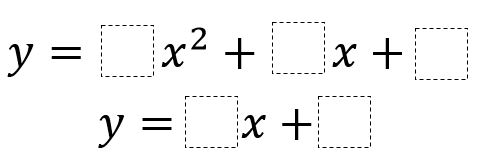Home > Calculus > Line Tangent to a Parabola

# Line Tangent to a Parabola

Directions: Use the digits 1 to 9, at most one time each, to fill in the boxes so that the line is tangent to the parabola.### Hint

Set the functions equal to each other. When solving this equation, the discriminant must be 0.